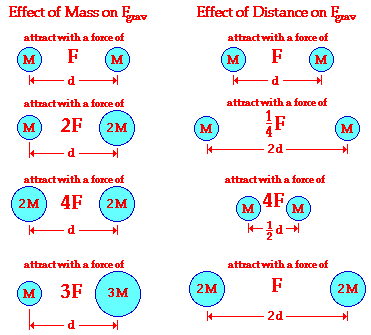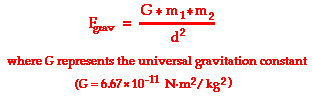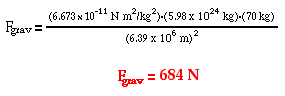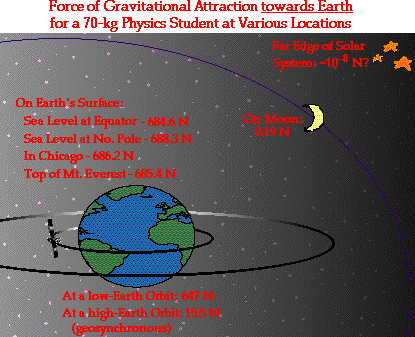Circular Motion and Satellite Motion - Lesson 3 - Universal Gravitation

# Newton's Law of Universal Gravitation

As discussed earlier in Lesson 3, Isaac Newton compared the acceleration of the moon to the acceleration of objects on earth. Believing that gravitational forces were responsible for each, Newton was able to draw an important conclusion about the dependence of gravity upon distance. This comparison led him to conclude that the force of gravitational attraction between the Earth and other objects is inversely proportional to the distance separating the earth's center from the object's center. But distance is not the only variable affecting the magnitude of a gravitational force. Consider Newton's famous equation

Fnet = m • a

Newton knew that the force that caused the apple's acceleration (gravity) must be dependent upon the mass of the apple. And since the force acting to cause the apple's downward acceleration also causes the earth's upward acceleration (Newton's third law), that force must also depend upon the mass of the earth. So for Newton, the force of gravity acting between the earth and any other object is directly proportional to the mass of the earth, directly proportional to the mass of the object, and inversely proportional to the square of the distance that separates the centers of the earth and the object.

### The UNIVERSAL Gravitation Equation

But Newton's law of universal gravitation extends gravity beyond earth. Newton's law of universal gravitation is about the universality of gravity. Newton's place in the Gravity Hall of Fame is not due to his discovery of gravity, but rather due to his discovery that gravitation is universal. ALL objects attract each other with a force of gravitational attraction. Gravity is universal. This force of gravitational attraction is directly dependent upon the masses of both objects and inversely proportional to the square of the distance that separates their centers. Newton's conclusion about the magnitude of gravitational forces is summarized symbolically asSince the gravitational force is directly proportional to the mass of both interacting objects, more massive objects will attract each other with a greater gravitational force. So as the mass of either object increases, the force of gravitational attraction between them also increases. If the mass of one of the objects is doubled, then the force of gravity between them is doubled. If the mass of one of the objects is tripled, then the force of gravity between them is tripled. If the mass of both of the objects is doubled, then the force of gravity between them is quadrupled; and so on.

Since gravitational force is inversely proportional to the square of the separation distance between the two interacting objects, more separation distance will result in weaker gravitational forces. So as two objects are separated from each other, the force of gravitational attraction between them also decreases. If the separation distance between two objects is doubled (increased by a factor of 2), then the force of gravitational attraction is decreased by a factor of 4 (2 raised to the second power). If the separation distance between any two objects is tripled (increased by a factor of 3), then the force of gravitational attraction is decreased by a factor of 9 (3 raised to the second power).

### Thinking Proportionally About Newton's Equation

The proportionalities expressed by Newton's universal law of gravitation are represented graphically by the following illustration. Observe how the force of gravity is directly proportional to the product of the two masses and inversely proportional to the square of the distance of separation.Another means of representing the proportionalities is to express the relationships in the form of an equation using a constant of proportionality. This equation is shown below.The constant of proportionality (G) in the above equation is known as the universal gravitation constant. The precise value of G was determined experimentally by Henry Cavendish in the century after Newton's death. (This experiment will be discussed later in Lesson 3.) The value of G is found to be

G = 6.673 x 10-11 N m2/kg2

The units on G may seem rather odd; nonetheless they are sensible. When the units on G are substituted into the equation above and multiplied by m1• m2 units and divided by d2 units, the result will be Newtons - the unit of force.

### Using Newton's Gravitation Equation to Solve Problems

Knowing the value of G allows us to calculate the force of gravitational attraction between any two objects of known mass and known separation distance. As a first example, consider the following problem.

 Sample Problem #1 Determine the force of gravitational attraction between the earth (m = 5.98 x 1024 kg) and a 70-kg physics student if the student is standing at sea level, a distance of 6.38 x 106 m from earth's center.

The solution of the problem involves substituting known values of G (6.673 x 10-11 N m2/kg2), m1 (5.98 x 1024 kg), m2 (70 kg) and d (6.38 x 106 m) into the universal gravitation equation and solving for Fgrav. The solution is as follows:Sample Problem #2 Determine the force of gravitational attraction between the earth (m = 5.98 x 1024 kg) and a 70-kg physics student if the student is in an airplane at 40000 feet above earth's surface. This would place the student a distance of 6.39 x 106 m from earth's center.

The solution of the problem involves substituting known values of G (6.673 x 10-11 N m2/kg2), m1 (5.98 x 1024 kg), m2 (70 kg) and d (6.39 x 106 m) into the universal gravitation equation and solving for Fgrav. The solution is as follows:Two general conceptual comments can be made about the results of the two sample calculations above. First, observe that the force of gravity acting upon the student (a.k.a. the student's weight) is less on an airplane at 40 000 feet than at sea level. This illustrates the inverse relationship between separation distance and the force of gravity (or in this case, the weight of the student). The student weighs less at the higher altitude. However, a mere change of 40 000 feet further from the center of the Earth is virtually negligible. This altitude change altered the student's weight changed by 2 N that is much less than 1% of the original weight. A distance of 40 000 feet (from the earth's surface to a high altitude airplane) is not very far when compared to a distance of 6.38 x 106 m (equivalent to nearly 20 000 000 feet from the center of the earth to the surface of the earth). This alteration of distance is like a drop in a bucket when compared to the large radius of the Earth. As shown in the diagram below, distance of separation becomes much more influential when a significant variation is made.The second conceptual comment to be made about the above sample calculations is that the use of Newton's universal gravitation equation to calculate the force of gravity (or weight) yields the same result as when calculating it using the equation presented in Unit 2:

Fgrav = m•g = (70 kg)•(9.8 m/s2) = 686 N

Both equations accomplish the same result because (as we will study later in Lesson 3) the value of g is equivalent to the ratio of (G•Mearth)/(Rearth)2.

### The Universality of Gravity

Gravitational interactions do not simply exist between the earth and other objects; and not simply between the sun and other planets. Gravitational interactions exist between all objects with an intensity that is directly proportional to the product of their masses. So as you sit in your seat in the physics classroom, you are gravitationally attracted to your lab partner, to the desk you are working at, and even to your physics book. Newton's revolutionary idea was that gravity is universal - ALL objects attract in proportion to the product of their masses. Gravity is universal. Of course, most gravitational forces are so minimal to be noticed. Gravitational forces are only recognizable as the masses of objects become large. To illustrate this, use Newton's universal gravitation equation to calculate the force of gravity between the following familiar objects. Click the buttons to check answers.

 Mass of Object 1 (kg) Mass of Object 2 (kg) Separation Distance (m) Force of Gravity (N) a. Football Player 100 kg Earth 5.98 x1024 kg 6.38 x 106 m (on surface) See AnswerFgrav = 980 N b. Ballerina 40 kg Earth 5.98 x1024 kg 6.38 x 106 m (on surface) See AnswerFgrav = 392 N c. Physics Student 70 kg Earth 5.98 x1024 kg 6.60 x 106 m (low-height orbit) See AnswerFgrav = 641 N d. Physics Student 70 kg Physics Student 70 kg 1 m See AnswerFgrav = 3.27 • 10-7 N e. Physics Student 70 kg Physics Student 70 kg 0.2 m See AnswerFgrav = 8.17 • 10-6 N f. Physics Student 70 kg Physics Book 1 kg 1 m See AnswerFgrav = 4.67 • 10-9 N g. Physics Student 70 kg Moon 7.34 x 1022 kg 1.71 x 106 m (on surface) See AnswerFgrav = 117 N h. Physics Student 70 kg Jupiter 1.901 x 1027 kg 6.98 x 107 m (on surface) See AnswerFgrav = 1823 N

Today, Newton's law of universal gravitation is a widely accepted theory. It guides the efforts of scientists in their study of planetary orbits. Knowing that all objects exert gravitational influences on each other, the small perturbations in a planet's elliptical motion can be easily explained. As the planet Jupiter approaches the planet Saturn in its orbit, it tends to deviate from its otherwise smooth path; this deviation, or perturbation, is easily explained when considering the effect of the gravitational pull between Saturn and Jupiter. Newton's comparison of the acceleration of the apple to that of the moon led to a surprisingly simple conclusion about the nature of gravity that is woven into the entire universe. All objects attract each other with a force that is directly proportional to the product of their masses and inversely proportional to their distance of separation.

### Investigate!

Use the Newton's Law of Universal Gravitation widget below to investigate the effect of the object masses and separation distance upon the amount of gravitational attraction. Enter the masses of the two objects and their separation distance. Then click the Submit button to view the gravitational force. Experiment with various values of mass and distance.
E

 How did Newton establish that it was the force of gravity between the sun and the planets was the force responsible for keeping the planets in motion along their elliptical path? Click to see.

### We Would Like to Suggest ...Sometimes it isn't enough to just read about it. You have to interact with it! And that's exactly what you do when you use one of The Physics Classroom's Interactives. We would like to suggest that you combine the reading of this page with the use of our Gravitation Interactive. You can find it in the Physics Interactives section of our website. The Gravitation Interactive allows a learner to interactively explore the inverse square law of gravitation.

### Check Your Understanding

1. Suppose that two objects attract each other with a gravitational force of 16 units. If the distance between the two objects is doubled, what is the new force of attraction between the two objects?

2. Suppose that two objects attract each other with a gravitational force of 16 units. If the distance between the two objects is reduced in half, then what is the new force of attraction between the two objects?

3. Suppose that two objects attract each other with a gravitational force of 16 units. If the mass of both objects was doubled, and if the distance between the objects remained the same, then what would be the new force of attraction between the two objects?

4. Suppose that two objects attract each other with a gravitational force of 16 units. If the mass of both objects was doubled, and if the distance between the objects was doubled, then what would be the new force of attraction between the two objects?

5. Suppose that two objects attract each other with a gravitational force of 16 units. If the mass of both objects was tripled, and if the distance between the objects was doubled, then what would be the new force of attraction between the two objects?

6. Suppose that two objects attract each other with a gravitational force of 16 units. If the mass of object 1 was doubled, and if the distance between the objects was tripled, then what would be the new force of attraction between the two objects?

7. As a star ages, it is believed to undergo a variety of changes. One of the last phases of a star's life is to gravitationally collapse into a black hole. What will happen to the orbit of the planets of the solar system if our star (the Sun shrinks into a black hole)? (And of course, this assumes that the planets are unaffected by prior stages of the Sun's evolving stages.)

8. Having recently completed her first Physics course, Dawn Well has devised a new business plan based on her teacher's Physics for Better Living theme. Dawn learned that objects weigh different amounts at different distances from Earth's center. Her plan involves buying gold by the weight at one altitude and then selling it at another altitude at the same price per weight. Should Dawn buy at a high altitude and sell at a low altitude or vice versa?

9. Anita Diet is very concerned about her weight but seldom does anything about it. After learning about Newton's law of universal gravitation in Physics class, she becomes all concerned about the possible effect of a change in Earth's mass upon her weight. During a (rare) free moment at the lunch table, she speaks up "How would my weight change if the mass of the Earth increased by 10%?" How would you answer Anita?

10. When comparing mass and size data for the planets Earth and Jupiter, it is observed that Jupiter is about 300 times more massive than Earth. One might quickly conclude that an object on the surface of Jupiter would weigh 300 times more than on the surface of the Earth. For instance, one might expect a person who weighs 500 N on Earth would weigh 150000 N on the surface of Jupiter. Yet this is not the case. In fact, a 500-N person on Earth weighs about 1500 N on the surface of Jupiter. Explain how this can be.

Next Section:
Jump To Next Lesson:

Follow Us